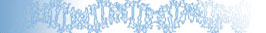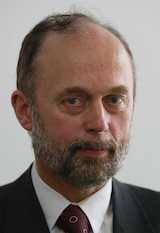## Prof Andrzej Piegat

Westpomeranian University of Technology, Poland

### Why multidimensional fuzzy arithmetic in problem solving

#### Abstract:

The aim of the talk is to familiarize conference participants with fuzzy arithmetic (in a general sense) and with fuzzy multivariate arithmetic, in order to show what kinds of problems can and should be solved with its use. In this talk, the authors will try to answer the following questions:

• What do you need fuzzy arithmetic for?
• Are conventional arithmetic and math enough to solve practical problems?
• Precise (ideal) data and approximate (real) data.
• An example illustrating the need to use FA- control of movement of Boeing 747 aircraft.
• Gradual development of fuzzy arithmetic
• Examples of existing types of fuzzy arithmetic
• Fuzzy 2-dimensional and multidimensional arithmetic.
• Type 0, Type 1, and Type 2- multidimensional fuzzy arithmetic.
• Result of using multidimensional fuzzy arithmetic to simulate airplane motion and other examples.#### Biography:

Andrzej Piegat received a Ph.D. degree in 1979 at the Szczecin University of Technology in the field of discrete production processes control. He obtained a D. Sc degree at the University of Rostock, Germany, in 1988 in the field of control of unmanned underwater vehicles. The title of professor he received from the President of Poland in 2001. In 1991 he began studies and scientific work in the field of Artificial Intelligence, mainly Fuzzy Systems. In 2001, he published the book "Fuzzy Modeling and Control" in the Springer-Verlag publishing house, which was also published in Russia and Poland. During the last 12 years he has been mainly involved in fuzzy arithmetic - he is the creator of the concept of multidimensional fuzzy arithmetic. Together with his colleagues, Marcin Plucinski and Marek Landowski have prepared over 40 articles in this field. He is currently preparing to write a book on the multidimensional fuzzy arithmetic of Type 0, Type 1, and Type 2.

Back to Keynote Speakers

KES-2021

25th Annual
KES Conference
Szczecin, Poland
8-10 September 2021